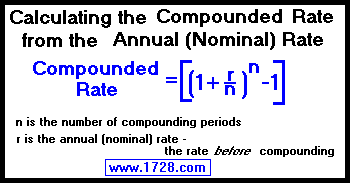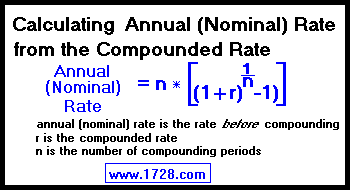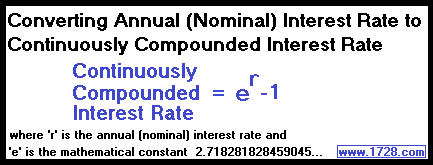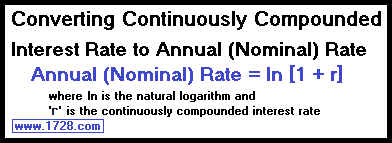Calculating Compound Interest Rates Revised March, 2014 annual (nominal) rate - Basically, this is the rate before it is compounded. compounded rate - Rate after it has been compounded.8 per cent interest compounded semi-annually equals what annual (nominal) rate? We know the annual (nominal) rate is 8 per cent so: Compounded Interest Rate = (1 + [.08 ÷ 2])2 -1 Compounded Interest Rate = (1 + .04)2 -1 Compounded Interest Rate = 1.0816 -1 Compounded Interest Rate = .0816 which equals 8.16 per cent. You probably have concluded that: n = 4 for quarterly compounded interest n = 12 for monthly compounded interest and n = 365 for daily compounded interest.If we have a monthly compounded interest rate of .072290080856235 (or 7.2290080856235%), what was the rate before compounding? (Or what is the annual (nominal) rate?)Since we are dealing with monthly compounding, n=12. Putting the numbers into the formula, we see that the annual (nominal) rate equals: 12 * [(1 + .072290080856235)(1 ÷ 12)-1)] = 12 * [(1.072290080856235)(.08333333...)-1)] = 12 * (1.00583333333333... -1) = 12 * (.00583333333333) annual (nominal) rate = .07 or 7 per cent Continuously Compounded InterestFor the first time, we have a formula that uses the mathematical constant e which equals 2.71828182845904523536.... So, let's comptue the continuously compounded rate of an annual (nominal) rate of 9 per cent. continuously compounded rate = er -1 continuously compounded rate = (2.71828...).09-1 continuously compounded rate = 1.09417428370521 -1 continuously compounded rate = .09417428370521 continuously compounded rate = 9.417428370521%A bank account yields 6% interest when compounded continuously. What is the annual (nominal) interest rate? annual (nominal) rate = natural log [1 + .06] annual (nominal) rate = 0.0582689081239758 rate = 5.82689081239758% before compounding